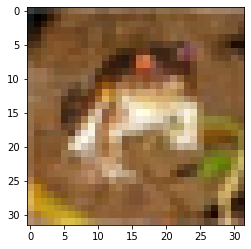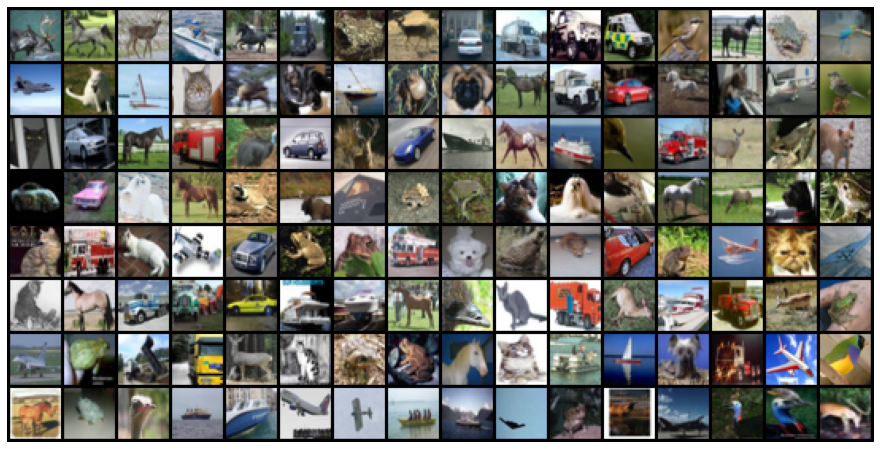abhinaykrishna-t17/03-cifar10-feedforward - Jovian
Learn data science and machine learning by building real-world projects on Jovian

Classifying images of everyday objects using a neural network

The ability to try many different neural network architectures to address a problem is what makes deep learning really powerful, especially compared to shallow learning techniques like linear regression, logistic regression etc.

In this assignment, you will:

1. Explore the CIFAR10 dataset: https://www.cs.toronto.edu/~kriz/cifar.html
2. Set up a training pipeline to train a neural network on a GPU
3. Experiment with different network architectures & hyperparameters

As you go through this notebook, you will find a ??? in certain places. Your job is to replace the ??? with appropriate code or values, to ensure that the notebook runs properly end-to-end. Try to experiment with different network structures and hypeparameters to get the lowest loss.

You might find these notebooks useful for reference, as you work through this notebook:

# Uncomment and run the commands below if imports fail
# !conda install numpy pandas pytorch torchvision cpuonly -c pytorch -y
# !pip install matplotlib --upgrade --quiet
import torch
import torchvision
import numpy as np
import matplotlib.pyplot as plt
import torch.nn as nn
import torch.nn.functional as F
from torchvision.datasets import CIFAR10
from torchvision.transforms import ToTensor
from torchvision.utils import make_grid
from torch.utils.data import random_split
%matplotlib inline
# Project name used for jovian.commit
project_name = '03-cifar10-feedforward'

Exploring the CIFAR10 dataset

test_dataset = CIFAR10(root='data/', train=False, transform=ToTensor())
HBox(children=(FloatProgress(value=1.0, bar_style='info', max=1.0), HTML(value='')))
Extracting data/cifar-10-python.tar.gz to data/

Q: How many images does the training dataset contain?

dataset_size = len(dataset)
dataset_size
50000

Q: How many images does the training dataset contain?

test_dataset_size = len(test_dataset)
test_dataset_size
10000

Q: How many output classes does the dataset contain? Can you list them?

Hint: Use dataset.classes

classes =dataset.classes
classes
['airplane',
'automobile',
'bird',
'cat',
'deer',
'dog',
'frog',
'horse',
'ship',
'truck']
num_classes = len(dataset.classes)
num_classes
10

Q: What is the shape of an image tensor from the dataset?

img, label = dataset
img_shape = img.shape
img_shape
torch.Size([3, 32, 32])

Note that this dataset consists of 3-channel color images (RGB). Let us look at a sample image from the dataset. matplotlib expects channels to be the last dimension of the image tensors (whereas in PyTorch they are the first dimension), so we'll the .permute tensor method to shift channels to the last dimension. Let's also print the label for the image.

img, label = dataset
plt.imshow(img.permute((1, 2, 0)))
print('Label (numeric):', label)
print('Label (textual):', classes[label])
Label (numeric): 6 Label (textual): frog(Optional) Q: Can you determine the number of images belonging to each class?

Hint: Loop through the dataset.

class_dict = {0:0,1:0,2:0,3:0,4:0,5:0,6:0,7:0,8:0,9:0}
def class_counter(class_dict,num):
class_dict[num] = class_dict[num] +1
return class_dict

for _,class_num in dataset:
class_dict = class_counter(class_dict,class_num)
class_dict
{0: 5000,
1: 5000,
2: 5000,
3: 5000,
4: 5000,
5: 5000,
6: 5000,
7: 5000,
8: 5000,
9: 5000}

Let's save our work to Jovian, before continuing.

import jovian
jovian.commit(project=project_name, environment=None)
[jovian] Detected Colab notebook... [jovian] Please enter your API key ( from https://jovian.ai/ ): API KEY: ·········· [jovian] Uploading colab notebook to Jovian... [jovian] Committed successfully! https://jovian.ai/abhinaykrishna-t17/03-cifar10-feedforward

Preparing the data for training

We'll use a validation set with 5000 images (10% of the dataset). To ensure we get the same validation set each time, we'll set PyTorch's random number generator to a seed value of 43.

torch.manual_seed(43)
val_size = 5000
train_size = len(dataset) - val_size

Let's use the random_split method to create the training & validation sets

train_ds, val_ds = random_split(dataset, [train_size, val_size])
len(train_ds), len(val_ds)
(45000, 5000)

We can now create data loaders to load the data in batches.

batch_size=128

Let's visualize a batch of data using the make_grid helper function from Torchvision.

print('images.shape:', images.shape)
plt.figure(figsize=(16,8))
plt.axis('off')
plt.imshow(make_grid(images, nrow=16).permute((1, 2, 0)))
break
images.shape: torch.Size([128, 3, 32, 32])Can you label all the images by looking at them? Trying to label a random sample of the data manually is a good way to estimate the difficulty of the problem, and identify errors in labeling, if any.

Base Model class & Training on GPU

Let's create a base model class, which contains everything except the model architecture i.e. it wil not contain the __init__ and __forward__ methods. We will later extend this class to try out different architectures. In fact, you can extend this model to solve any image classification problem.

def accuracy(outputs, labels):
_, preds = torch.max(outputs, dim=1)
class ImageClassificationBase(nn.Module):
def training_step(self, batch):
images, labels = batch
out = self(images)                  # Generate predictions
loss = F.cross_entropy(out, labels) # Calculate loss
return loss

def validation_step(self, batch):
images, labels = batch
out = self(images)                    # Generate predictions
loss = F.cross_entropy(out, labels)   # Calculate loss
acc = accuracy(out, labels)           # Calculate accuracy
return {'val_loss': loss.detach(), 'val_acc': acc}

def validation_epoch_end(self, outputs):
batch_losses = [x['val_loss'] for x in outputs]
epoch_loss = torch.stack(batch_losses).mean()   # Combine losses
batch_accs = [x['val_acc'] for x in outputs]
epoch_acc = torch.stack(batch_accs).mean()      # Combine accuracies
return {'val_loss': epoch_loss.item(), 'val_acc': epoch_acc.item()}

def epoch_end(self, epoch, result):
print("Epoch [{}], val_loss: {:.4f}, val_acc: {:.4f}".format(epoch, result['val_loss'], result['val_acc']))

We can also use the exact same training loop as before. I hope you're starting to see the benefits of refactoring our code into reusable functions.

outputs = [model.validation_step(batch) for batch in val_loader]
return model.validation_epoch_end(outputs)

history = []
optimizer = opt_func(model.parameters(), lr)
for epoch in range(epochs):
# Training Phase
loss = model.training_step(batch)
loss.backward()
optimizer.step()
# Validation phase
model.epoch_end(epoch, result)
history.append(result)
return history

Finally, let's also define some utilities for moving out data & labels to the GPU, if one is available.

torch.cuda.is_available()
False
def get_default_device():
"""Pick GPU if available, else CPU"""
if torch.cuda.is_available():
else:
device = get_default_device()
device
device(type='cpu')
def to_device(data, device):
"""Move tensor(s) to chosen device"""
if isinstance(data, (list,tuple)):
return [to_device(x, device) for x in data]
return data.to(device, non_blocking=True)

"""Wrap a dataloader to move data to a device"""
def __init__(self, dl, device):
self.dl = dl
self.device = device

def __iter__(self):
"""Yield a batch of data after moving it to device"""
for b in self.dl:
yield to_device(b, self.device)

def __len__(self):
"""Number of batches"""
return len(self.dl)

Let us also define a couple of helper functions for plotting the losses & accuracies.

def plot_losses(history):
losses = [x['val_loss'] for x in history]
plt.plot(losses, '-x')
plt.xlabel('epoch')
plt.ylabel('loss')
plt.title('Loss vs. No. of epochs');
def plot_accuracies(history):
accuracies = [x['val_acc'] for x in history]
plt.plot(accuracies, '-x')
plt.xlabel('epoch')
plt.ylabel('accuracy')
plt.title('Accuracy vs. No. of epochs');

Let's move our data loaders to the appropriate device.